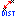# Geometric Distance

This category contains 8 nodes.

##### Distance (1-D)Streamable

This node calculates the distance between two points in 1-D space

##### Distance (2-D)Streamable

This node calculates the distance between two points in 2-D space

##### Distance (3-D)Streamable

This node calculates the distance between two points in 3-D space

##### Distance (n-D)Streamable

This node calculates the distance between two points in n-D space

##### Vector Distance (1-D)Streamable

This node calculates the vector distance between two points in 1-D space

##### Vector Distance (2-D)Streamable

This node calculates the vector distance between two points in 2-D space

##### Vector Distance (3-D)Streamable

This node calculates the vector distance between two points in 3-D space

##### Vector Distance (n-D)Streamable

This node calculates the vector distance between two points in n-D space